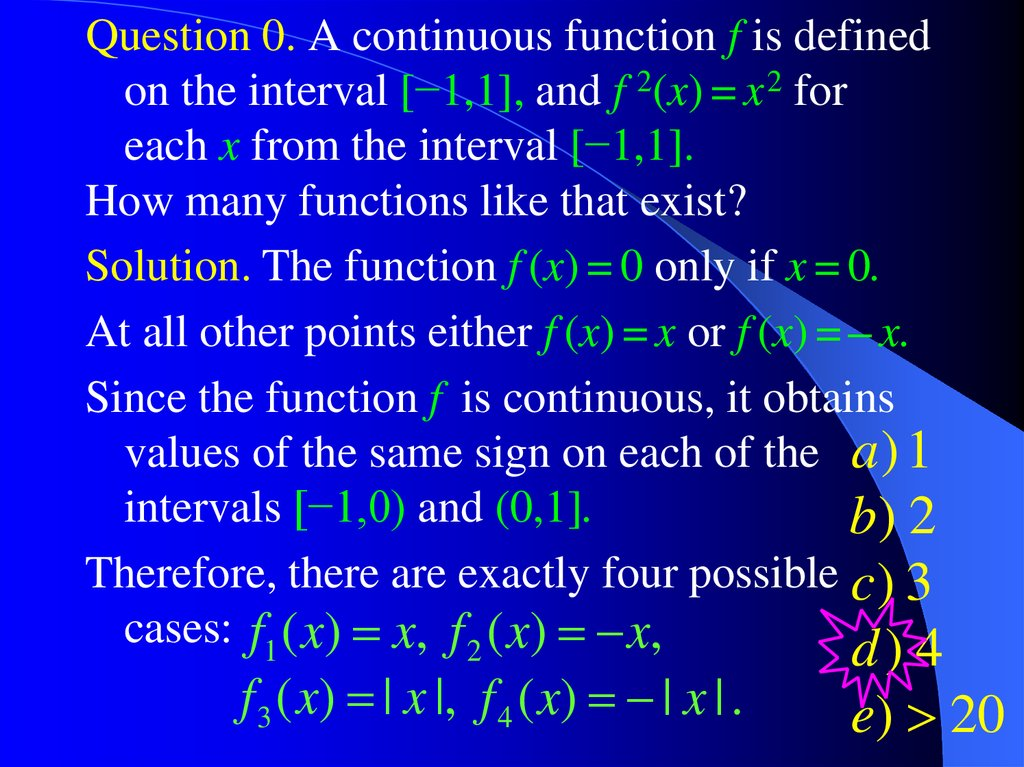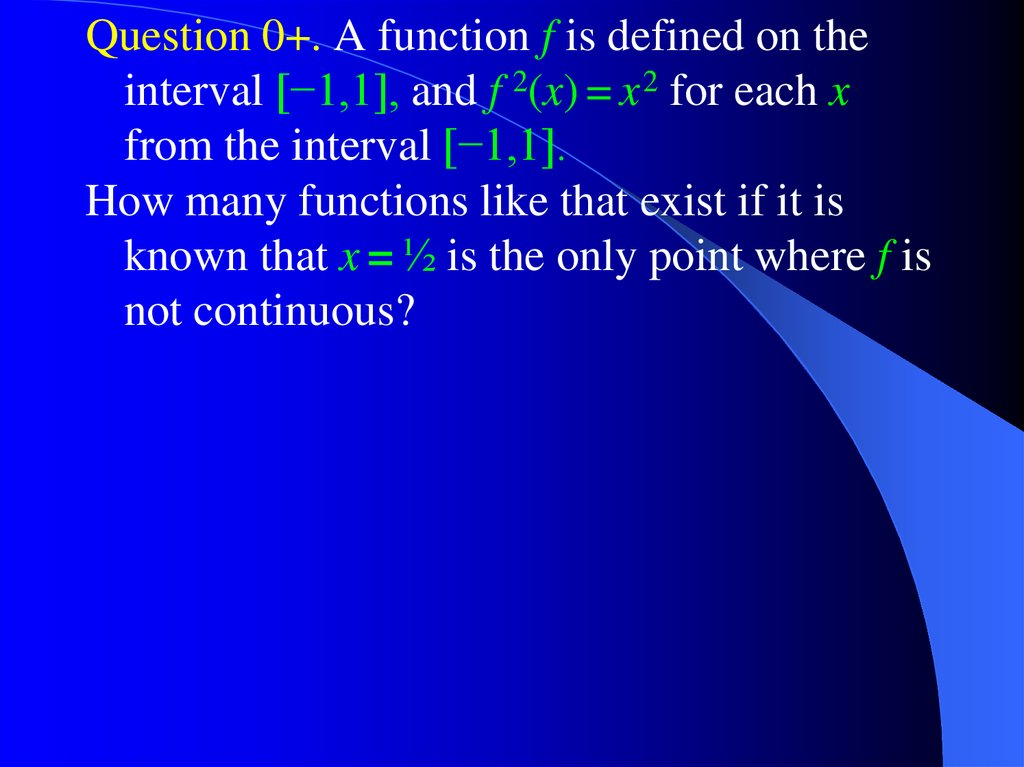# Mathematical Induction

Calculus++ Light

## 2.

Question 0. A continuous function f is defined
on the interval [−1,1], and f 2(x) = x 2 for
each x from the interval [−1,1].
How many functions like that exist?
Solution. The function f (x) = 0 only if x = 0.
At all other points either f (x) = x or f (x) = – x.
Since the function f is continuous, it obtains
values of the same sign on each of the a ) 1
intervals [−1,0) and (0,1].
b) 2
Therefore, there are exactly four possible c ) 3
cases: f1 ( x) x, f 2 ( x) x,
f 3 ( x) | x |, f 4 ( x) | x | .
d) 4
e) 20

## 3.

Question 0+. A function f is defined on the
interval [−1,1], and f 2(x) = x 2 for each x
from the interval [−1,1].
How many functions like that exist if it is
known that x = ½ is the only point where f is
not continuous?

## 4. Mathematical Induction

Let Sn, n = 1,2,3,… be statements involving
positive integer numbers n.
Suppose that
1. S1 is true.
2. If Sk is true, then Sk +1 is also true.
Then Sn is true for all positive integer numbers n.

## 5.

Question 1. Using the Principle of
Mathematical Induction show that
n(n 1)( 2n 1)
for
any
n
=
1,2,3,….
m
6
m 1
n
2
Solution. Step 1. The formula is correct in the
1(1 1)( 2 1)
case n = 1, because 1
.
6
2
Step 2. Let as assume that the formula is
k (k 1)( 2k 1)
correct for n = k: m
.
6
m 1
k
2
Our aim is to show, that in this case the
formula is also correct for n = k + 1.

## 6.

k 1
We have
k
m m
2
m 1
2
(k 1)
2
m 1
k (k 1)( 2k 1)
2
(k 1)
6
k (2k 1)
(k 1)
k 1
6
2
2k 7 k 6
(k 2)( 2k 3)
(k 1)
(k 1)
6
6
(k 1)(( k 1) 1)( 2(k 1) 1)
.
6
Now, the principle of mathematical induction
tells us that our formula is correct for any n.

## 7.

Answers to Questions from Light #3:
Functions and Limits
Question 2: e) the open first quadrant
Question 3: lim x
x 0
ln1x
1
e .
Question 4: e) I and III
Question 5: b) a nonconstant function
Question 6: f (x) is positive for all x > 0

## 8.

Calculus++
Also known as
Hysterical Calculus

## 9.

Question 1b. Using the Principle of
Mathematical Induction show that
sin( 2n x)
cos x cos(3x) cos(( 2n 1) x)
.
2
sin
x
for any n = 1,2,3,….
Solution. Step 1. The formula is correct in the
case n = 1, because sin( 2 x) 2 sin x cos x.
sin( 2 x)
And hence cos x
.
2 sin x
Step 2. Let as assume that the formula is
correct for n = k:
sin( 2k x)
cos x cos(3x) cos(( 2k 1) x)
.
2 sin x

## 10.

Our aim is to show, if our formula is correct
for n = k, then it is also correct for n = k + 1.
cos x cos(3x) cos(( 2k 1) x)
sin( 2k x)
cos(( 2k 1) x)
cos(( 2k 1) x)
2 sin x
sin( 2k x) 2 sin x cos(( 2k 1) x)
2 sin x
sin( 2k x) sin(( 2k 1) x x) sin(( 2k 1) x x)
2 sin x
sin( 2(k 1) x)
2 sin x
Now, the principle of mathematical induction
tells us that our formula is correct for any n.

## 11.

Question 3a. Calculate the following sum
n
mq
m
.
m 0
Solution. We have
n 1
d 1 q
d
m
mq q q q
dq 1 q
dq m 0
m 0
n 1
n 1
(1 q ) (1 q) (1 q )(1 q)
q
2
(
1
q
)
n
n 1
(n 1)q (1 q) (1 q )
q
2
(1 q)
n 1
n
1 nq (n 1)q
q
2
(1 q)
n
m
n

## 12.

Question 5. Using the formula for the
derivative of inverse function derive explicit
formulae for the derivatives of arcsin x,
arccos x, arctan x, and arccot x.
1
Solution. Using the formula g ( x)
f ( y ) y g ( x )
we obtain the following formula for the
derivative of arcsin x:
d
1
1
arcsin x
.
dx
(sin y ) y arcsin x cos y y arcsin x
The range of arcsin x is the interval [ 12 , 12 ].
Therefore cos(arcsin x) is always non-negative.

## 13.

Hence
1
d
1
.
arcsin x
2
2
dx
1 x
1 sin (arcsin x)
Similar calculations yield the following
formula for the derivative of arccot x.
d
1
2
arccot x
sin y
y arccot x
dx
(cot y ) y arccot x
1
1
2
2
1 cot y y arccot x
1 cot (arccot x)
1
.
2
1 x

## 14.

Question 6. Use the Cauchy criterion to show
n
1
that the sequence xn 2 , n 1,2, ,
k 1 k
converges.
Solution: It is sufficient to show that the
sequence xn is fundamental:
0, N , n N , m 0 : xn m xn .
n m
n m
1
1
We have xn m xn 2
k n 1 k
k n 1 ( k 1) k
n m
1 1
1
1
1
1
k n n 1 n 1 n 2
k n 1 k 1
1
1
1
1
n m 1 n m
n 2 n 3

## 15.

m
1
1
1
.
n n m n ( n m) n
1
Thus xn m xn .
n
1
Therefore 0, N , we set N
,
n N , m 0 :
1 1
1
xn m xn 1 .
n N
Thus, the sequence xn is fundamental, and
therefore it converges to some limit L.
In fact, L
2
6
.

## 16. Picture of the Week

All ICEF students are of the
same height

## 17.

Question 4. Let f (x) be a differentiable
function such that the derivative f (x) is a
continuous function and f (f (x)) = x for any
x. Furthermore, let f (0) = 1, and f (1) = 0.
a) Is it possible that there exists a number a
such that f (a) 0 ?
Solution: Differentiate the equation f (f (x)) = x
to obtain f ( f ( x)) f ( x) 1.
Therefore, there are no points a such that
f (a) 0,
because at a point like that
f ( f (a)) f (a) 0 1.

## 18.

b) Is it possible that there exists a number a
such that f (a) 0 ?
Solution: The Mean Value Theorem tells us
that
f (1) f (0) 0 1
f (c)
1 0
1 0
1.
for some point c: 0 < c < 1.
Therefore, there are no points a such that
f (a) 0,
otherwise there would be a point b
somewhere between c and a such that
f (b) 0.

## 19.

c) Let x1 be a solution of the equation f (x) = x.
Find f ( x1 ).
Solution: If f (x1) = x1, then
1 ( f ( f ( x))) x x f ( f ( x1 )) f ( x1 )
1
f ( x1 ) f ( x1 ) f ( x1 ) 1.

## 20.

Answers to Questions from Seminar 3.
1
a
Questions 1a: lim xn b .
n
Questions 2a: 2
1 ln 2
2
Questions 7: c) I and II only
Question 8a: yn 3 yn 2 yn 1 12 yn 32 .
3
Question 8b: lim yn .
n
7 1
Question 8c: xn 2 xn 1 2 xn 54 , lim xn 12 .
n
yn 2 yn 1 12 yn 12 , lim yn 15 .
n